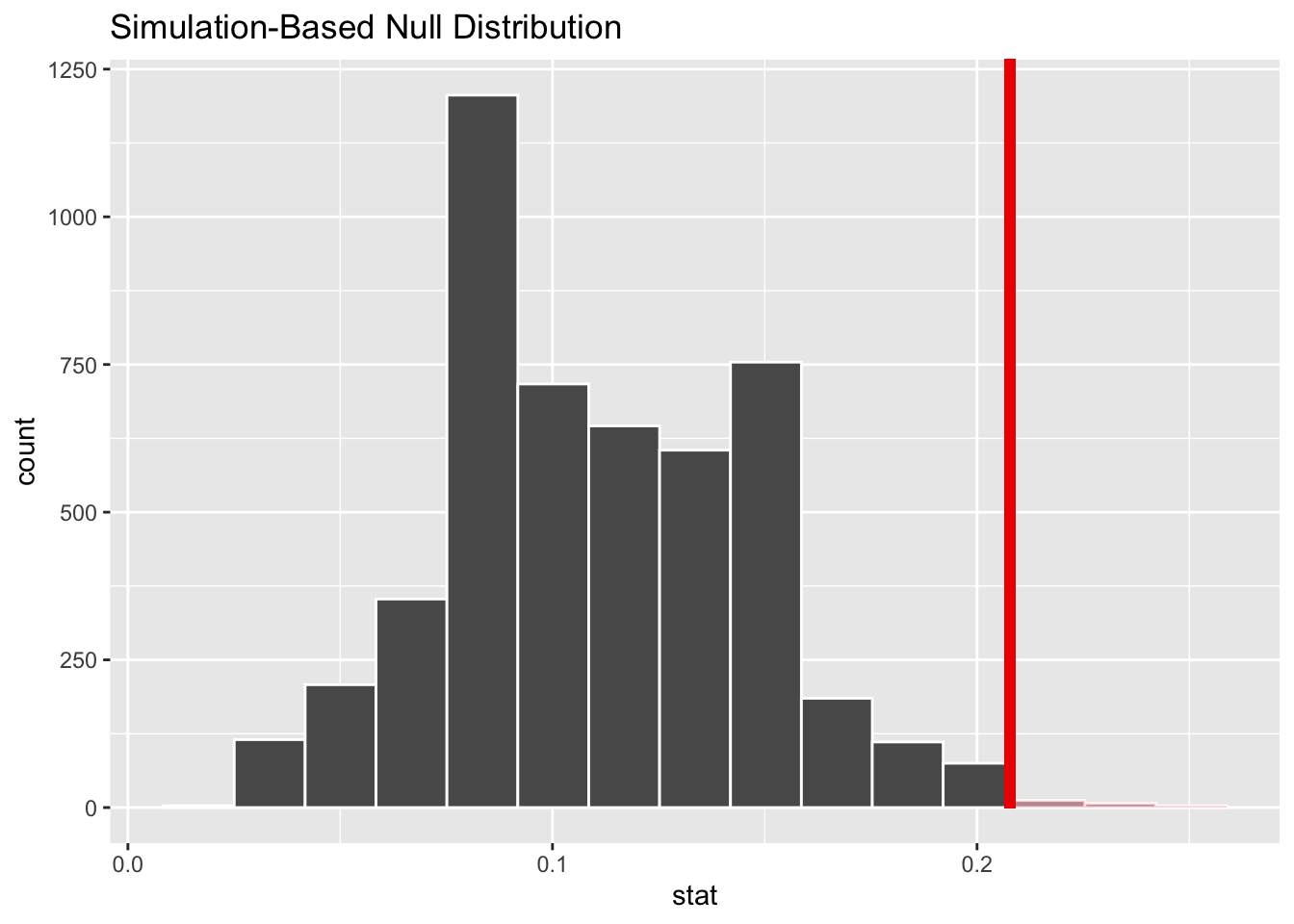# Packages and data

library(tidyverse)
library(infer)
hand <- read_csv("data/handedness.csv")

# Data

hand_prop <- hand %>%
count(handedness) %>%
mutate(proportion = n / sum(n)) %>%
pull(proportion)

hand_prop
##  0.2077922

# Exercises

The handedness dataset contains a random sample of 77 Duke students with respect to their handedness status (one mixed-handed student was asked to choose which hand they primarily used to brush their teeth). It is documented that approximately 11 percent of the world is left-handed (Hardyck and Petrinovich, 1977 Psychological Bulletin). You are interested in whether Duke has a higher proportion of left-handed students than would be expected from the world average.

1. Write out your null and alternative hypotheses in both words and symbols, being sure to define any notation used.

$H_0: p = 0.11$ $H_1: p > 0.11$

$$H_0$$ the proportion of students at Duke that are left-handed is equal to the world average of 0.11.

$$H_1$$: the proportion of students at Duke that are left-handed is greater than the world average of 0.11.

1. Conduct a simulation-based test of your hypotheses in Exercise 1, using 5,000 simulations (set a random seed of 12345) and report your p-value.
set.seed(12345)
null_dist <- hand %>%
specify(response = handedness, success = "left") %>%
hypothesize(null = "point", p = .11) %>%
generate(reps = 5000, type = "simulate") %>%
calculate(stat = "prop")
null_dist %>%
visualise() +
shade_p_value(obs_stat = hand_prop, direction = "greater")null_dist %>%
filter(stat >= hand_prop) %>%
summarise(p_value = n() / nrow(null_dist))
null_dist %>%
get_p_value(obs_stat = hand_prop, direction = "greater")
1. What do you conclude in the context of your research question?

Let $$\alpha = 0.01$$.

Since the p-value is less than $$\alpha$$, we reject the null hypothesis at the 0.01 significance level. We have enough strong enough evidence to suggest that the true population proportion of Duke students that are left-handed is not equal to 0.11 at the 0.01 significance level.